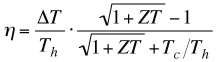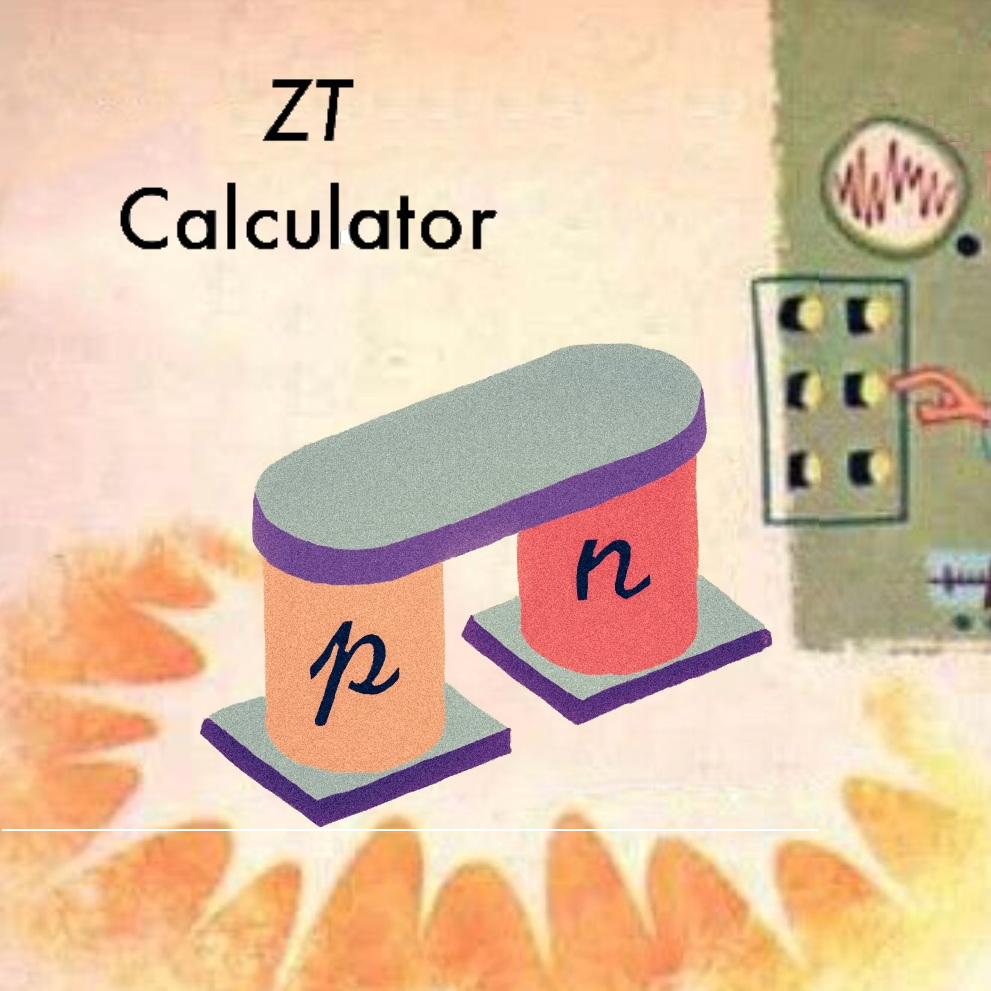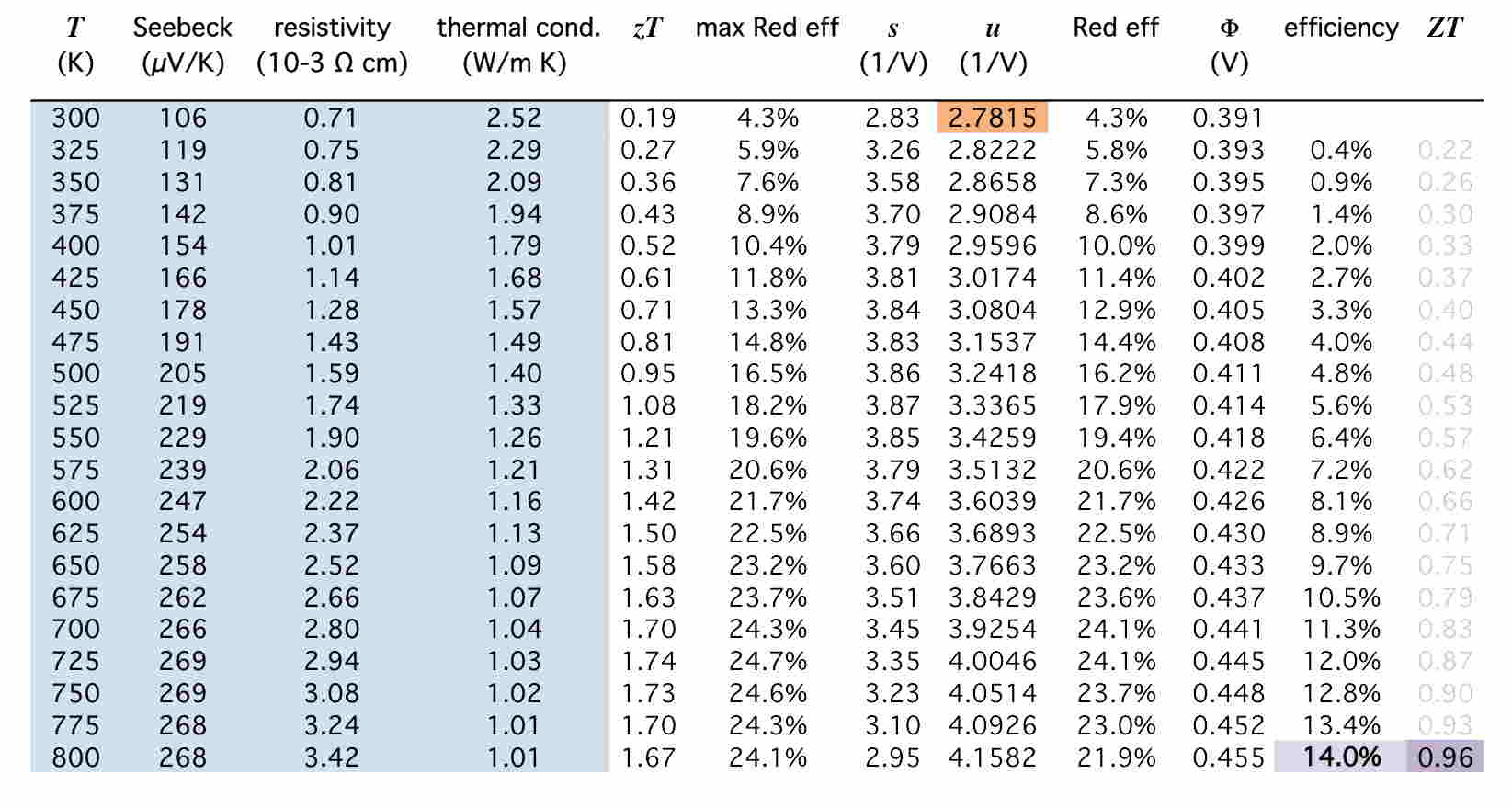# Procedure to Calculate Device ZT from materials properties

The efficiency of a Thermoelectrc Device is typically reported as a function of ZT (Device Figure of Merit)Here we show you how to calculate ZT from thermoelectric materials properties that are temperature dependent as described in . You will need the thermoelectric properties of your material as a function of temperature from Tc (cold side temperature) to Th (hot side temperature).

## Automated Web version of the caclulator ready for initial testingIt should look like the figure below.There are two worksheets with one p-type example and one n-type example.

The necessary formulas are simple and should be readable by any spreadsheet program that reads *.xls files.

Dont worry if the figures or plots dont work in your spreadsheet program they are not essential for the calculation. If you prefer a python program try this at GitHub.

## 1. Put data into spreadsheet caclulator.

The first column is the Temperature (in K). You will replace this column with the temperatures of your thermoelectric data points. Put in your temperature dependent Seebeck coefficient (in micro-Volts/K), resistivity (in milli-Ohm * cm), and thermal conductivity (in W/mK) into the appropriate columns. Replace everything in the first 4 columns in the table (shaded light blue). You can use more or fewer rows of data than that in the example.

The cold side temperature for the calculation is the first temperature, 300K in the example. We shall use 800K for hot side temperature for the example. The example shows 25K temperature intervals between data points, but that can vary. You can use smaller temperature steps for more accurate calculations.

## 2. Optimize efficiency by changing the first u value

Adjust the first entry of the relative current value u that is shaded orange in the Spreadsheet. You can start with value similar to the s values in the column to the left of u. The values for u at higher temperature will adjust accordingly – don't change these.

Maximize the efficiency at the hot side - the last entry in the efficiency column (shaded light purple in the example spreadsheet). You can do this by hand or with the solver tool in MS Excel [Solver Help]

## 3. Record your ZT value

Once you have maximized the efficiency at Th, you can read off the device ZT from the column to the right - shaded dark purple.

ZT is calculated from the maximum efficiency value you found by optimizing cold side u value. In the spreadsheet example ZT = 0.96 for the temperature range between 300K and 800K. For ZT at other Th or Tc, the u value at Tc will need to be reoptimized. For example this same file can be used to calculate ZT = 0.72 for the 300K-600K temperature range (not ZT = 0.66 in Table I as that u was optimized for 300K-800K)

## References

The following journal article gives a complete description of the calculation for reference

 G. J. Snyder, A. H. Snyder "Figure of Merit ZT of a Thermoelectric Device Defined from Materials Properties" Energy and Environmental Science 10, 2280 (2017)

Further details and derivation of the calculations can be found in

 G. J. Snyder "Thermoelectric Power Generation: Efficiency and Compatibility" Chapter 9, in Thermoelectrics Handbook: Macro to Nano edited by D. M. Rowe, CRC Press (2006).

 A python and Java Script version of the calculator written by A. H. Snyder can be downloaded from GitHub. Link to the JavaScript version of the calculator.